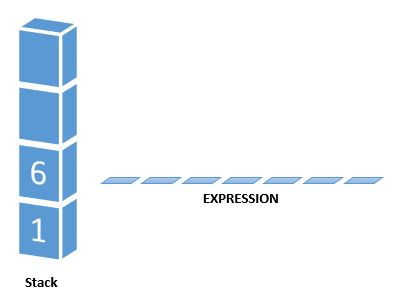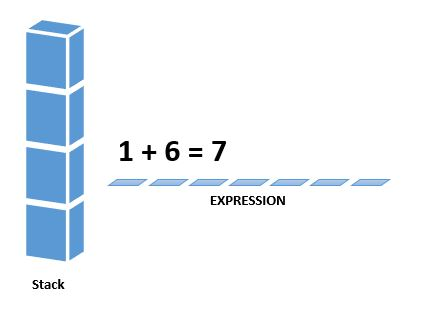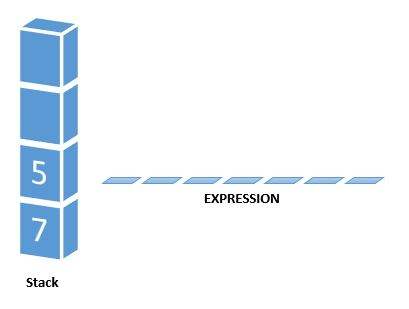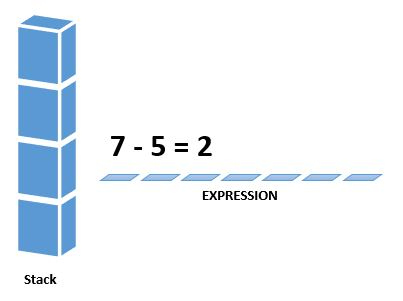Home Theory Discuss Postfix Evaluation using stacks

# Discuss Postfix Evaluation using stacks

1 comment

Post-fix expressions have a very special place in computer science. Most of the computations are performed using stacks. In-fact a very simple calculator works on the principle of post-fix evaluation to get the answer. A post-fix notation is also known as the reverse Polish notation. The steps involved in evaluating a post-fix expression can be defined as follows. We use the concept of stacks to evaluate the same.

#### ALGORITHM:

1. Scan the post-fix string from left to right.
2. Initialize an empty stack.
3. Repeat the below steps 4 and 5 till all the characters are scanned.
4. If the scanned character is an operand, push it onto the stack.
5. If the scanned character is an operator, and
• If the operator is a unary operator then pop an element from the stack.
• If the operator is binary operator then pop two elements from the stack.AFTER POPPING, APPLY THE OPERATOR TO THOSE POPPED ELEMENTS AND PUSH THE RESULT IN THE STACK.
6. After all the characters are scanned, we will have only one element in the stack.
7. This only element is the result of the expression.

#### EXAMPLE:

Let us see how the above algorithm works with the help of an example.
Our sample post-fix string 1 2 3 * + 5 –

1. Initially our stack is empty. Now the first three characters are scanned 1, 2 and 3 which are operands. They will be pushed into the stack in that order.2. Next character scanned is ” * “, which is an operator. Thus, we pop the top two elements from the stack and perform the * operation with the two operands. The second operand will be the first element that is popped.3. The value of the expression (2 * 3) that has been evaluated (6) is pushed into the stack.4. Next character scanned is ” + “ which is an operator. Thus, we pop the two elements from the stack and perform the “+” operation with the two operands. Again remember, the second operand will be the first element that is popped.5. The value of the expression (1 + 6) has been evaluated (7) and has been pushed into the stack.6. Next character scanned is “5”, which is added to the stack.7. Next character scanned is ” – “, which is an operator. Thus, we pop the top two elements from the stack and perform the “-” operation with the two operands. The second operand will be the first element that is popped.8. The value of the expression (7 – 5) has been evaluated (2) and is pushed into the stack.Now since we are out of characters to be scanned, we are left with only one value in the stack. That means that our answer to the post-fix expression is 2.

#### 1 commentNovember 13, 2016 - 08:36

nice bro, but include some complex examples……